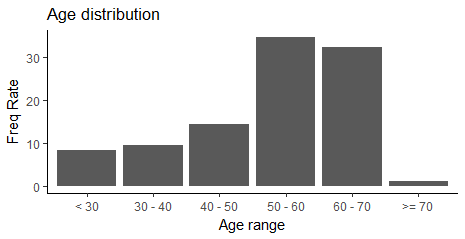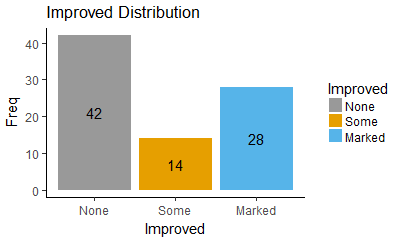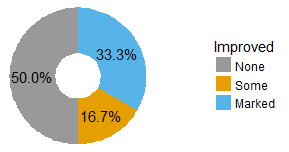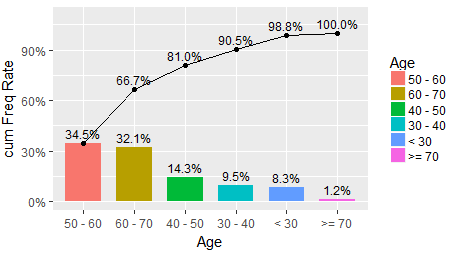数据分析 第三篇：数据特征分析（分布+帕累托+周期）

library(grid)
library(vcd)

ID Treatment  Sex Age Improved
1 57   Treated Male  27     Some
2 46   Treated Male  29     None
3 77   Treated Male  30     None
4 17   Treated Male  32   Marked
5 36   Treated Male  46   Marked
6 23   Treated Male  58   Marked

一，定量数据的分布分析

• 求值域（range）：值域 = 最大值 - 最小值
• 决定组距和组数：组距是每个区间的长度，组数 = 值域 /  组距
• 决定组限： 组限是指每个区间的端点，这一步是要确定每组的起点和终点
• 列出频率分布表
• 绘制频率分布直方图

• 各组之间是互斥的
• 各组的组距相等

（1）制作频率分布表

library(grid)
library(vcd)

labels <- c("< 30", "30 - 40", "40 - 50", "50 - 60", "60 - 70", ">= 70")
breaks <- c(1,30,40,50,60,70,100)
mytable <- cut(Arthritis$Age, breaks = breaks, labels = labels, right = TRUE ) df <- as.data.frame(table(Age=mytable)) df <- transform(df, cumFreq = cumsum(Freq), FreqRate = prop.table(Freq)) df <- transform(df, CumFreqRate= cumsum(FreqRate)) df <- transform(df,FreqRate=round(FreqRate * 100,2), CumFreqRate= round(CumFreqRate*100,2)) （2）绘制频率分布直方图 使用ggplot绘制频率分布直方图： ggplot(data=df, mapping=aes(x=factor(Age),y=FreqRate,group=factor(Age))) + geom_bar(stat="identity")+ labs(title='Age distribution',x='Age range',y='Freq Rate')+ theme_classic()二，定性数据的分布分析 对于定性变量，通常根据分类来分组，然后统计分组的频数或频率，可以采用饼图或条形图来描述定性数据的分布： • 饼图的每一个扇形部分代表每一类型的百分比或频数，根据定性变量的类型把饼图分成几个部分，每一个部分的大小与每一个类型的频数成正比； • 条形图的高度代表每一类型的百分比或频数，条形图的宽度没有意义。 按照Improved变量的频数来绘制饼图和条形图： mytable <- with(Arthritis, table(Improved)) df <- as.data.frame(mytable) 1，绘制条形图 使用geom_bar绘制条形图： ggplot(data=df,mapping = aes(x=Improved, y=Freq,fill=Improved)) + geom_bar(stat="identity")+ scale_fill_manual(values=c("#999999", "#E69F00", "#56B4E9"))+ labs(title='Improved Distribution', x='Improved',y='Freq')+ geom_text(stat="identity",aes(y=Freq, label = Freq), size=4, position=position_stack(vjust = 0.5))+ theme_classic()2，绘制饼图 使用geom_bar()和 coord_polar() 函数来绘制饼图，通常情况下，饼图显示的是百分比，而直方图显示的某个分类的具体数值： blank_theme <- theme_minimal()+ theme( axis.title.x = element_blank(), axis.title.y = element_blank(), axis.text.x = element_blank(), axis.text.y = element_blank(), panel.border = element_blank(), panel.grid=element_blank(), axis.ticks = element_blank(), plot.title=element_text(size=14, face="bold") ) ggplot(data=df, mapping=aes(x="Improved",y=Freq,fill=Improved))+ geom_bar(stat="identity",width=0.5,position='stack',size=5)+ coord_polar("y", start=0)+ scale_fill_manual(values=c("#999999", "#E69F00", "#56B4E9"))+ blank_theme + geom_text(stat="identity",aes(y=Freq, label = scales::percent(Freq/sum(Freq))), size=4, position=position_stack(vjust = 0.5))三，帕累托分析 帕累托分析依据的原理是20/80定律，80%的效益常常来自于20%的投入，而其他80%的投入却只产生了20%的效益，这说明，同样的投入在不同的地方会产生不同的效益。 怕累托图的绘制过程是按照贡献度从高到低依次排列，并绘制累积贡献度曲线。当样本数量足够大时，贡献度通常会呈现20/80分布。 使用ggplot2绘制的帕累托图的脚本和图如下所示：library(grid) library(vcd) library(ggplot2) library(scales) labels <- c("< 30", "30 - 40", "40 - 50", "50 - 60", "60 - 70", ">= 70") breaks <- c(1,30,40,50,60,70,100) mytable <- cut(Arthritis$Age, breaks = breaks, labels = labels, right = TRUE )
df <- as.data.frame(table(Age=mytable),stringsAsFactors=FALSE)
df <- transform(df, FreqRate = prop.table(Freq))

df <- df[order(df$Freq,decreasing =TRUE),] rownames(df) <- seq(nrow(df)) df$Age <- factor(df$Age,levels=df$Age)
df$cumRate <- cumsum(df$FreqRate)
df$cumRateLable <- as.character(percent(df$cumRate))

df\$cumRateLable <- ""

ggplot(df, aes(x=Age,y=FreqRate,fill=Age)) +
geom_bar(stat="identity",width = 0.7) +
geom_text(stat='identity',aes(label=percent(FreqRate)),vjust=-0.5, color="black", size=3)+
scale_y_continuous(name="cum Freq Rate",limits=c(0, 1.1),labels = function(x) paste0(x*100, "%"))+
geom_point(aes(y=cumRate),show.legend=FALSE) +
geom_text(stat="identity",aes(label=cumRateLable,y=cumRate), vjust=-0.5, size=3)+
geom_path(aes(y=cumRate, group=1))
View Code

四，周期性分析

posted @ 2018-08-20 14:20  悦光阴  阅读(7003)  评论(0编辑  收藏  举报